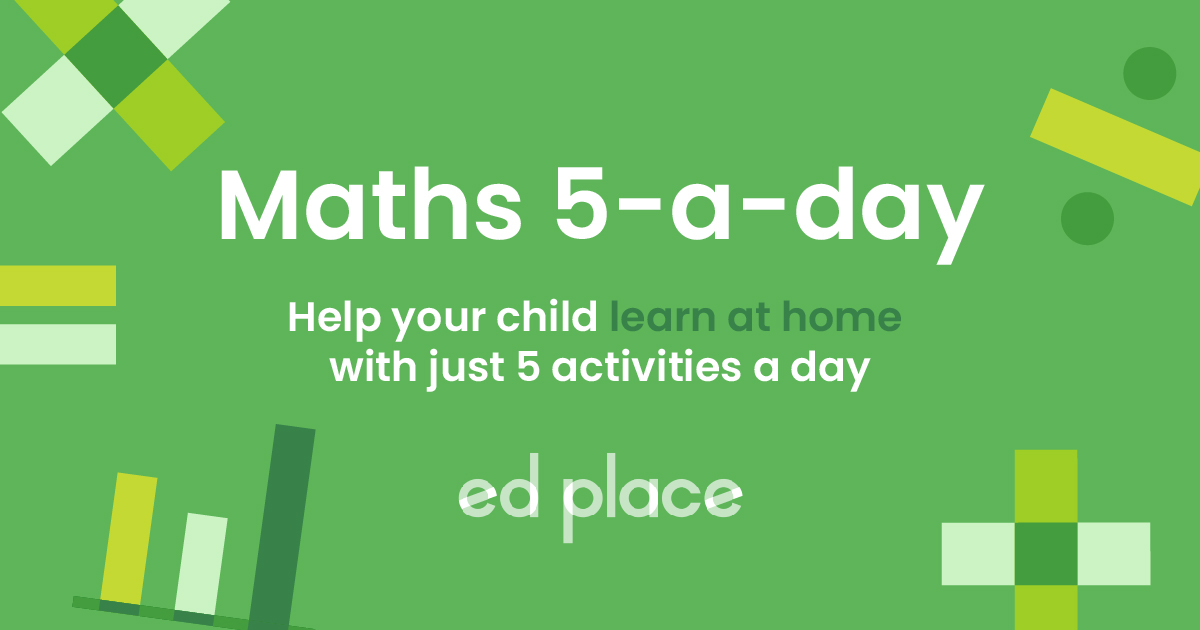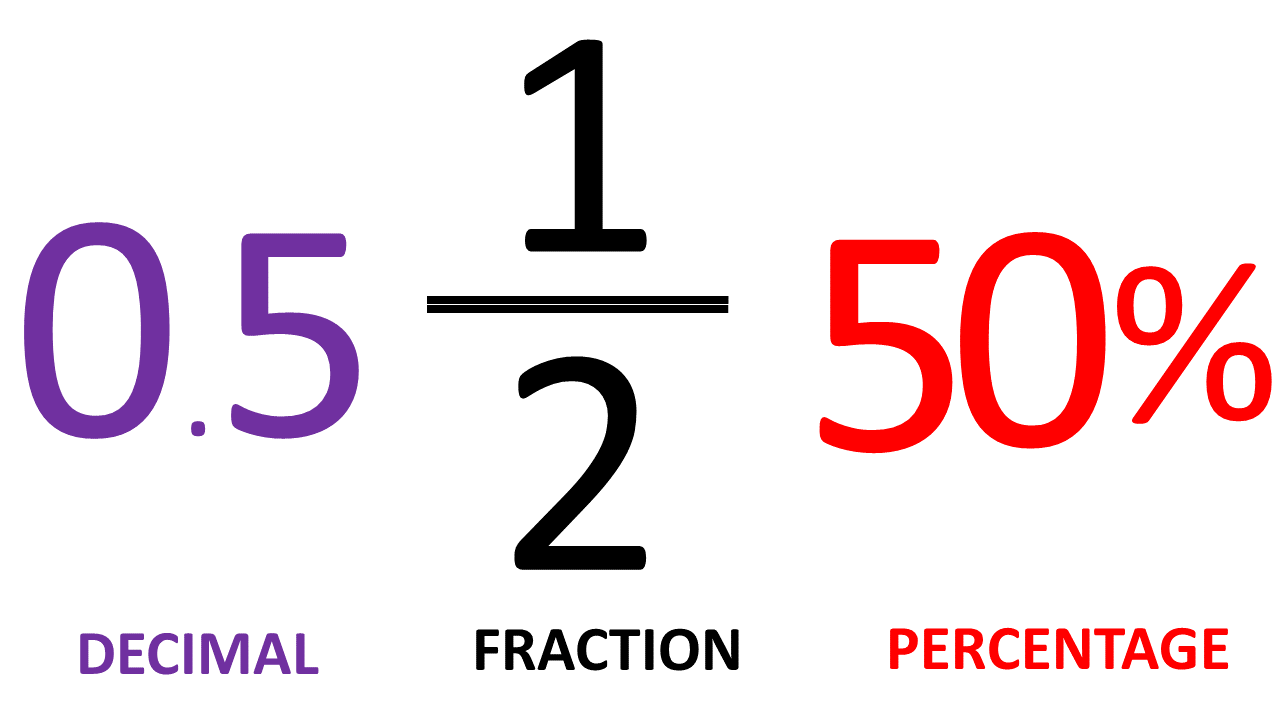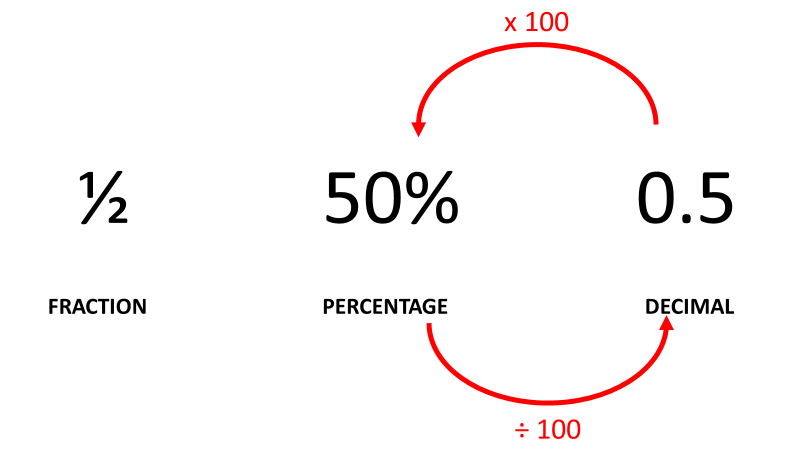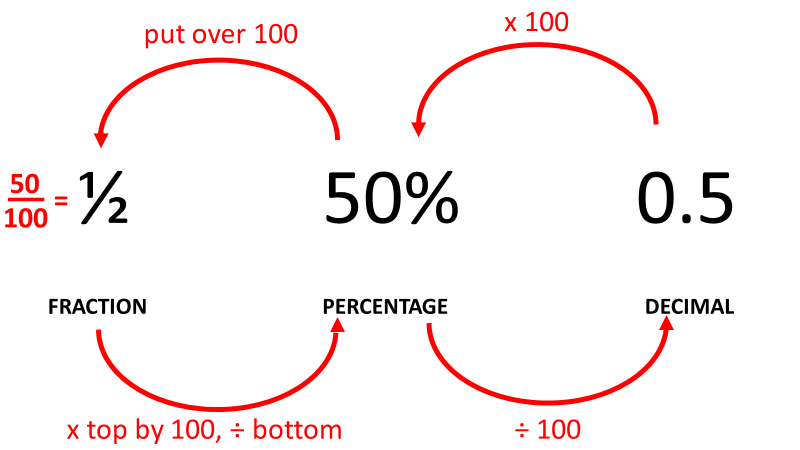# EdPlace's KS3 home learning maths lesson: Converting Between Fractions, Decimals and Percentages

Looking for short lessons to keep your child engaged and learning? Our experienced team of teachers have created English, maths and science lessons for the home, so your child can learn no matter where they are.  And, as all activities are self-marked, you really can encourage your child to be an independent learner.

Get them started on the lesson below and then jump into our teacher-created activities to practice what they've learnt. We've recommended five to ensure they feel secure in their knowledge - 5-a-day helps keeps the learning loss at bay (or so we think!).

Are they keen to start practising straight away? Head to the bottom of the page to find the activities.

Now...onto the lesson!

Key Stage 3 statutory requirements for maths
Year 7 students should be able to interpret percentages and percentage changes as a fraction or a decimal.

# Calculating Quantities...

In Year 7, students will be fairly comfortable when it comes to working with different quantities.  But, it's really important that they are able to understand how a quantity in the format of a fraction can be converted to a decimal or percentage.  Being able to convert between fractions, decimals, and percentages is an essential skill at Key Stage 3 as it greatly develops a student’s concept of what the quantities mean. It also makes fractions and percentages of amounts much simpler to visualise and compare.

We're confident that if you follow the step-by-step approach below you'll have a 100% success rate:

1) Convert between fractions, decimals and percentages

2) Simplify quantities

3) Arrange a combination of these in order

## Defining the Quantities

Before you begin, it helps for students to know how we define the key quantities that we will be discussing.

Percentage  - this means 'out of 100'

Fraction -  a number less than 1

Decimal - a number whose 'whole' part and 'fractional' part is separated by a decimal point

## Step 1 - Same Thing... Different Presentation

Begin by showing your child these three quantities and ask if they are different from each other, or the same thing.They should confidently know that ½, 50%, and 0.5 represent the same thing, just in three different forms. Then ask: “how can you prove it?”  This may lead to your child attempting to draw pie charts or number lines or struggling to put it into words. Explain that the method you are about to see will teach a very simple way of converting between all three formats – and it will work every time.

## Step 2 - Converting Between Percentages and Decimals

The most obvious conversion is between percentages and decimals. We can see that 50% and 0.5 differ by just a couple of decimal places. It's important to point out that this is a factor of a 100 as many students make the mistake of thinking it's 10.So, to turn a percentage to a decimal, divide by 100 and o turn a decimal to a percentage, multiply by 100.

## Step 3 - Percentages to Fractions

The next one is perhaps less obvious. How is 50% related to ½? The way to teach this is to explain that “percent” means “for every hundred”. Once that concept is understood, it should become obvious that percentages are just fractions of 100.To turn a percentage to a fraction, put it over 100. The reason 50/100 becomes ½ is that we've simplified it (this is a skill that should have been learned in Key Stage 2).

To turn a fraction into a percentage, we multiply the top by 100, then divide by the bottom. In the example we are doing: ½ = 100/2 = 50.

## Step 4 - Putting it All Together

The way to summarise these rules is to explain that it is all based around “100”. All we are really doing in each conversion is multiplying by 100 or dividing by 100.

One last handy shortcut to go over is how to turn a fraction directly into a decimal: divide the top by the bottom. A key concept that should be understood is the line in a fraction literally means “divide”. (e.g. 1/2 = 1 ÷ 2).

This is best demonstrated with a calculator:

½ = 1 ÷ 2 = 0.5

To turn decimals back into fractions, the simplest thing to do is turn them into percentages first, then put them over 100.

Now, you've followed the whole process through why not follow this approach through with these quantities?

a) 0.3

b) 0.25

c) 55%

d) 78%

e) 1/5

f) 1/8

## Challenge

One final thing to teach on this topic is how to apply this skill to arrange a set of numbers. For example, you may be asked to arrange the following from smallest to biggest:

## ¼, 3/8, 2/5, 0.72, 0.6, 0.525

It looks complicated, and, just as in Step 1, your child may find themselves trying to sketch pie charts or number lines. But, there's no need to do this as we've demonstrated. The easiest thing to do is to turn them all into percentages.  By converting them all into percentages, we can re-write the list:

## 25%, 37.5%, 40%, 72%, 60%, 52.5%

Suddenly it is a simple case of ordering a list of numbers with values between 0 and 100. The answer is of course:

## 25%, 37.5%, 40%, 52.5%, 60%, 72%

Something to emphasise here is 0.6 is greater than 0.525. Many students would look at the decimals and say: “but five hundred and twenty-five is greater than six!”

This is why we read the decimals as individual numbers (0.525 is pronounced “zero point five two five”, not “zero point five hundred and twenty-five”). This is an important point to reinforce, as it is a very common misconception. If a student doesn't read the decimal parts of a number correctly, they will go through Key Stage 3 thinking that having more decimal places automatically makes a number bigger, but it doesn’t.

## Step 5 - Give it a go...

Now see if you can tackle these activities!

All activities are created by teachers and automatically marked. Plus, with an EdPlace subscription, we can automatically progress your child at a level that's right for them. Sending you progress reports along the way so you can track and measure progress, together - brilliant!

a) 0.3 = 3/10, 30%

b) 0.25 = 1/4, 25%

c) 55% = 0.55, 11/20

d) 78% = 0.78, 39/50

e) 1/5 = 0.2, 20%

f) 1/8 = 0.125, 12.5%

Keep going! Looking for more activities, different subjects or year groups?

Click the button below to view the EdPlace English, maths, science and 11+ activity library